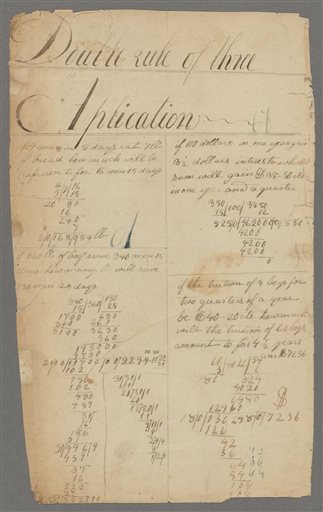# Lincoln's math problem

Alignments to Content Standards: 7.RP.A.3

If 100 dollars in one year gain $3\frac12$ dollars interest, what sum will gain \$38.50 cents in one year and a quarter? ## IM Commentary The purpose of this task is for students to solve a multi-step problem involving simple interest. What is most interesting about this task is that it was one that Abraham Lincoln worked on in his youth (probably around the age of 17 years); it was discovered in some old papers that were authenticated as Lincoln's. Here are some statements by Ken Clements, one of the researchers who analyzed the papers: Any student doing such work in the 1820s would have been more advanced than most on the frontier in Indiana. If you got to that stage, you'd sort of done well. The researchers also said that Lincoln is known to have later studied trigonometry and geometry on his own. You can read more about this research here. The problem as written in Lincoln's notebook does not explicitly say that students should assume simple interest, so if there is some question, the teacher can state this explicitly for them.## Solutions Solution: Lincoln's solution Here is the page containing his work:Here is his solution:Unfortunately, this is the largest image that could be found of the solution. Solution: A modern way of looking at Lincoln's solution to this problem Lincoln multiplied 3850, 12, and 100, then divided that by 350 times 15. Why does that work? \$38.50 is 3850 cents. One year is 12 months. $3\frac12$ dollars is 350 cents. One year and a quarter is 15 months.

The amount of interest 1 dollar would earn in 1 year is

$$\frac{350}{100}$$

Which is 3.5 cents. The amount of interest 1 dollar would earn in 1 month would be $\frac{1}{12}$ of that:

$$\frac{350}{100\times 12}$$

(Rather than compute this, let's leave it in this form so we can analyze the operations Lincoln did.)

To find the amount of money $x$ dollars would earn in 1 month, we should multiply the monthly rate by $x$:

$$x \cdot \frac{350}{100\times 12}$$

If we multiply that by 15 months, we know we will get $3850$ cents. So

$$x \cdot \frac{15\times350}{12\times 100}=3850$$

and

$$x = \frac{12\times 100\times3850}{15\times350} = (12\times 100\times3850)\div(15\times350)$$

Which is what Lincoln did. The amount is \$880. Solution: Another way of solving it If we multiply the principal by the yearly interest rate and the number of years, we will find the interest earned: $$I=Prt$$ The yearly interest rate is $$r=\frac{3.5}{100}=0.035$$ The number of years is 1.25 and the interest earned is 38.5. So: $$38.5=P\cdot 0.035\cdot 1.25$$ and $$P=38.5\div(0.035\cdot 1.25) = 880$$ So \$880 will earn \\$38.50 in a year and a quarter at the same rate.